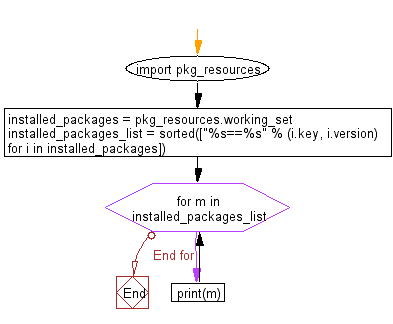﻿ Python: Get a list of locally installed Python modules - w3resource

# Python: Get a list of locally installed Python modules

## Python Basic - 1: Exercise-9 with Solution

Write a Python program to get a list of locally installed Python modules.

Sample Solution:

Python Code:

``````import pkg_resources
installed_packages = pkg_resources.working_set
installed_packages_list = sorted(["%s==%s" % (i.key, i.version)
for i in installed_packages])
for m in installed_packages_list:
print(m)
``````

Sample Output:

```asn1crypto==0.24.0
beautifulsoup4==4.5.1
biopython==1.71
bkcharts==0.2
bokeh==0.12.6
cairocffi==0.9.0
cairosvg==2.0.3
certifi==2018.11.29
cffi==1.11.5
chardet==3.0.4
cryptography==2.4.2
cssselect==1.0.1
cvxopt==1.2.2
cycler==0.10.0
decorator==4.3.0
ete3==3.1.1
genshi==0.7
html5lib==0.999
idna==2.8
jinja2==2.10
jsonschema==2.6.0
kiwisolver==1.0.1
lxml==3.7.0
markupsafe==1.1.0
marshmallow-polyfield==3.2
marshmallow==2.18.0
matplotlib==2.2.3
mpmath==1.1.0
mysql-connector-python==8.0.12
names==0.3.0
networkx==2.2
nose==1.3.7
ntlm-auth==1.2.0
numexpr==2.4.3
numpy==1.15.4
oauthlib==3.0.0
pandas==0.23.4
patsy==0.4.1
pillow==5.4.1
pip==18.0
ply==3.11
pronouncing==0.1.5
protobuf==3.6.1
psutil==5.4.8
pycep-correios==2.3.1
pycolors==0.1.2
pycparser==2.19
pycurl==7.43.0
pygal-maps-ch==1.0.1
pygal-maps-fr==1.1.0
pygal-maps-world==1.0.2
pygal==2.3.1
pygobject==3.20.0
pyparsing==2.3.1
python-apt==1.1.0b1+ubuntu0.16.4.1
python-dateutil==2.7.5
pytz==2018.9
pyyaml==3.13
qiskit-aer==0.1.0
qiskit-aqua==0.4.1
qiskit-terra==0.7.0
qiskit==0.7.0
requests-ntlm==1.1.0
requests-oauthlib==0.8.0
requests==2.21.0
scikit-learn==0.20.0
scipy==1.2.0
seaborn==0.8.1
setuptools==40.6.3
six==1.12.0
statsmodels==0.8.0
sympy==1.3
tables==3.2.2
tinycss==0.4
urllib3==1.24.1
wheel==0.29.0
```

Flowchart:Python Code Editor :

Have another way to solve this solution? Contribute your code (and comments) through Disqus.

What is the difficulty level of this exercise?

Test your Programming skills with w3resource's quiz.

﻿

## Python: Tips of the Day

Kwargs:

**kwargs and *args are function arguments that can be very useful.

They are quite underused and often under-understood as well.

Let's try to explain what kwargs are and how to use them.

• While *args are used to pass arguments at an unknown amount to functions, **kwargs are used to do the same but with named arguments.
• So, if *args is a list being passed as an argument, you can think of **kwargs as a dictionary that's being passed as an argument to a function.
• You can use arguments as you wish as long as you follow the correct order which is: arg1, arg2, *args, **kwargs. It's okay to use only one of those but you can't mix the order, for instance, you can't have: function(**kwargs, arg1), that'd be a major faux pas in Python.
• Another example: You can do function(*args,**kwargs) since it follows the correct order.
• Here is an example. Let's say satelites are given with their names and weight in tons in dictionary format. Code prints their weight as kilograms along with their names.
```def payloads(**kwargs):
for key, value in kwargs.items():
print( key+" |||", float(value)*100)
payloads(NavSat1 = '2.5', BaysatG2 = '4')
```

Output:

```NavSat1 ||| 250.0
BaysatG2 ||| 400.0```

Since the function above would work for any number of dictionary keys, **kwargs makes perfect sense rather than passing arguments with a fixed amount.

```def payloads(**kwargs):
for key, value in kwargs.items():
print( key+" |||", float(value)*100)

sats={"Tx211":"3", "V1":"0.50"}
```Tx211 ||| 300.0